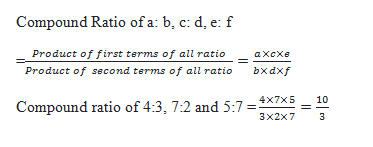# SSC CGL 2018 Practice Test Papers | Quantitative Aptitude (Day-49)

Dear Aspirants, Here we have given the Important SSC CGL Exam 2018 Practice Test Papers. Candidates those who are preparing for SSC CGL 2018 can practice these questions to get more confidence to Crack SSC CGL 2018 Examination.

[WpProQuiz 3528]

Click “Start Quiz” to attend these Questions and view Explanation

1) In a village, number of male, female and children are in the ratio of 3:5:7. If 90% of the males are literate, 80% of females are literate and 40% of children are illiterate, find the number of percentage of illiterate persons in the village.

a) 27.33%

b) 22%

c) 25%

d) 28%

2) A man deposited Rs. x at the rate of S.I in the beginning of first year and he increased his deposit by Rs. x at the beginning of every year. After 3yrs he got total amount Rs. 10368. If the rate of interest is 12% per annum then find the value of x.

a) 14400

b) 12000

c) 13000

d) 15500

3) In a school XYZ, there are 30 more girls than boys in class X. If the number of boys is increased by 20% and the number of girls is also increased by 10%, there would be 24 more girls than boys. What is the number of students in the school?

a) 210

b) 200

c) 180

d) 190

4) A circle whose area is 5544 sq. cm has circumference equal to the perimeter of a rectangle of length 75 cm. Find the area of rectangle.

a) 4575

b) 4275

c) 4485

d) None of these

5)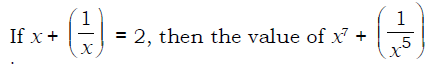a) 2

b) 12

c) 24

d) 29

6)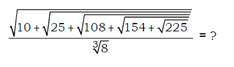a) 2

b) 4

c) 3

d) 5

7) Anand had 500 mangoes of which 20% were rotten. Earlier, Anand had planned to sell each mango at 20% above the cost price. Now, he removed the rotten mangoes and sold the remaining at 50% above the planned selling price. Find his overall profit percentage?

a) 24 %

b) 44%

c) 20 %

d) 32 %

8)  In an examination marks of P, Q and R, P scored 20% less than Q and scored 33 1/3 % less than R. Find the percent by which Q’s score must increase so that he scores as many marks as R.

a) 25%

b) 33 1/3 %

c) 20 %

d) None of these

9) A car travels x km at 60 km/hr and another 3x km at 90 km/hr. Find its average speed over the entire distance.

a) 84 km/hr

b) 75 km/hr

c) 72 km/hr

d) 80 km/hr

10) Find the compound ratio of 4: 3, 7:2 and 5:7 is

a) 10:3

b) 11: 17

c) 7: 9

d) None of these

Let the number of male female and children in the village be 3x, 5x and 7x respectively.

Total number of persons = 3x+ 5x+ 7x = 15x

Number of illiterate males = (100 – 90) % × 3x

= 10/100 ×3x

= 3x/10

Number of illiterate females = (100 – 80) % × 5x

= 20/100 ×5x

= x

Number of illiterate children = 40% × 7x

= 40/100 ×7x

= 28x/10

Number of illiterate persons

= 3x/10+ x+ 28x/10

= 41x/100

Percentage of illiterates = (41x/10)/(15x)x100= 27.33%

Principal= x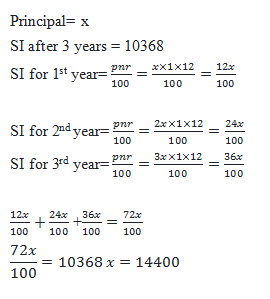Let there be ‘a’ boys and ‘b’ girls

b – a = 30—- (i)

110/100*b – 120/100*a = 24

1.1b-1.2a=24—– (ii)

Solving (i) and (ii) a = 90, b = 120

Total Students = a + b = 90 + 120 = 210

Area of circle= πr2 = 22/7×r×r = 5544

R = 42

Circumference of circle = 2πr = 2×22/7×42 = 264

Circumference of circle = (Perimeter of rectangle)

Perimeter of rectangle = 264

2(l + b) = 264

2(75+b) = 264

75+b =132

B = 57 cm

Area of Rectangle = lb

=75×57 = 4275 sq.cm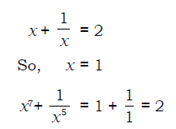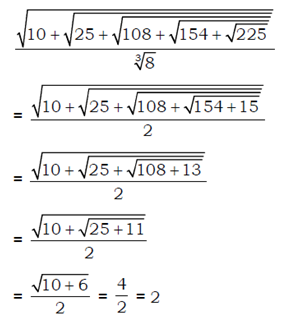Let the CP of each mango be Rs.10

So CP of 500 mangoes = 500×10 = 5000

20% mangoes are rotten.  Number of remaining mangoes = 80/100×500 = 400

At first he planned to sell the mangoes at 120/100×10 = Rs.12

New Selling Price = 150/100×12 = Rs. 18

SP of Remaining 400 mangoes = 400×18=7200

Profit = 7200 – 5000 = 2200

Profit Percentage = 2200/5000×100 = 44%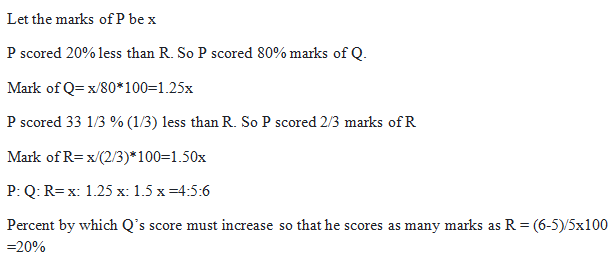D1= x km

S1= 60 km/hr

T1=distance/speed

= x/60

D2 = 3x

S2 = 90 km/hr

T2 = x/30 = x/60

Total Time = x/30+ x/60 = x/20

Total Distance = 3x+ x = 4x

Average Speed = 4x/(x/20) = 80 km/hr